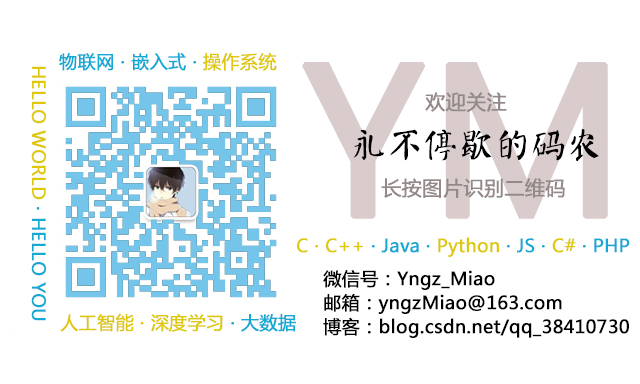# 【C++】C++11的std::function和std::bind用法详解

32 篇文章 17 订阅

## 可调用对象

C++中有如下几种可调用对象：函数、函数指针、lambda表达式、bind对象、函数对象。其中，lambda表达式和bind对象是C++11标准中提出的(bind机制并不是新标准中首次提出，而是对旧版本中bind1st和bind2st的合并)。个人认为五种可调用对象中，函数和函数指针本质相同，而lambda表达式、bind对象及函数对象则异曲同工。

### 函数指针

• 函数指针指向的是函数而非对象。和其他指针类型一样，函数指针指向某种特定类型；
• 函数类型由它的返回值和参数类型决定，与函数名无关
例如：
bool fun(int a, int b)


# include <iostream>

int fun(int x, int y) {                         //被调用的函数
std::cout << x + y << std::endl;
return x + y;
}

int fun1(int (*fp)(int, int), int x, int y) {   //形参为函数指针
return fp(x, y);
}

typedef int (*Ftype)(int, int);                 //定义一个函数指针类型Ftype
int fun2(Ftype fp, int x, int y) {
return fp(x, y);
}

int main(){
fun1(fun, 100, 100);                          //函数fun1调用函数fun
fun2(fun, 200, 200);                          //函数fun2调用函数fun
}


yngzmiao@yngzmiao-virtual-machine:~/test$g++ main.cc -o main -std=C++11 yngzmiao@yngzmiao-virtual-machine:~/test$ ./main
200
400


### lambda表达式

lambda表达式就是一段可调用的代码。主要适合于只用到一两次的简短代码段。由于lambda是匿名的，所以保证了其不会被不安全的访问:

# include <iostream>

int fun3(int x, int y){
auto f = [](int x, int y) { return x + y; };  //创建lambda表达式,如果参数列表为空，可以省去()
std::cout << f(x, y) << std::endl;            //调用lambda表达式
}

int main(){
fun3(300, 300);
}


### bind对象

std::bind可以用来生产，一个可调用对象来适应原对象的参数列表。具体的内容会在下文讲解。

## std::function

std::function是一个可调用对象包装器，是一个类模板，可以容纳除了类成员函数指针之外的所有可调用对象，它可以用统一的方式处理函数、函数对象、函数指针，并允许保存和延迟它们的执行

# include <functional>
std::function<函数类型>


# include <iostream>
# include <functional>

typedef std::function<int(int, int)> comfun;

// 普通函数
int add(int a, int b) { return a + b; }

// lambda表达式
auto mod = [](int a, int b){ return a % b; };

// 函数对象类
struct divide{
int operator()(int denominator, int divisor){
return denominator/divisor;
}
};

int main(){
comfun b = mod;
comfun c = divide();
std::cout << a(5, 3) << std::endl;
std::cout << b(5, 3) << std::endl;
std::cout << c(5, 3) << std::endl;
}


std::function可以取代函数指针的作用，因为它可以延迟函数的执行，特别适合作为回调函数使用。它比普通函数指针更加的灵活和便利。

1. std::function对C++中各种可调用实体(普通函数、Lambda表达式、函数指针、以及其它函数对象等)的封装，形成一个新的可调用的std::function对象，简化调用
2. std::function对象是对C++中现有的可调用实体的一种类型安全的包裹(如：函数指针这类可调用实体，是类型不安全的)

## std::bind

std::bind可以看作一个通用的函数适配器，它接受一个可调用对象，生成一个新的可调用对象来适应原对象的参数列表

std::bind将可调用对象与其参数一起进行绑定，绑定后的结果可以使用std::function保存。std::bind主要有以下两个作用：

• 将可调用对象和其参数绑定成一个仿函数
• 只绑定部分参数，减少可调用对象传入的参数

auto newCallable = bind(callable, arg_list);


#include <iostream>
#include <functional>

class A {
public:
void fun_3(int k,int m) {
std::cout << "print: k = "<< k << ", m = " << m << std::endl;
}
};

void fun_1(int x,int y,int z) {
std::cout << "print: x = " << x << ", y = " << y << ", z = " << z << std::endl;
}

void fun_2(int &a,int &b) {
++a;
++b;
std::cout << "print: a = " << a << ", b = " << b << std::endl;
}

int main(int argc, char * argv[]) {
//f1的类型为 function<void(int, int, int)>
auto f1 = std::bind(fun_1, 1, 2, 3); 					//表示绑定函数 fun 的第一，二，三个参数值为： 1 2 3
f1(); 													//print: x=1,y=2,z=3

auto f2 = std::bind(fun_1, std::placeholders::_1, std::placeholders::_2, 3);
//表示绑定函数 fun 的第三个参数为 3，而fun 的第一，二个参数分别由调用 f2 的第一，二个参数指定
f2(1, 2);												//print: x=1,y=2,z=3

auto f3 = std::bind(fun_1, std::placeholders::_2, std::placeholders::_1, 3);
//表示绑定函数 fun 的第三个参数为 3，而fun 的第一，二个参数分别由调用 f3 的第二，一个参数指定
//注意： f2  和  f3 的区别。
f3(1, 2);												//print: x=2,y=1,z=3

int m = 2;
int n = 3;
auto f4 = std::bind(fun_2, std::placeholders::_1, n); //表示绑定fun_2的第一个参数为n, fun_2的第二个参数由调用f4的第一个参数（_1）指定。
f4(m); 													//print: a=3,b=4
std::cout << "m = " << m << std::endl;					//m=3  说明：bind对于不事先绑定的参数，通过std::placeholders传递的参数是通过引用传递的,如m
std::cout << "n = " << n << std::endl;					//n=3  说明：bind对于预先绑定的函数参数是通过值传递的，如n

A a;
//f5的类型为 function<void(int, int)>
auto f5 = std::bind(&A::fun_3, &a, std::placeholders::_1, std::placeholders::_2); //使用auto关键字
f5(10, 20);												//调用a.fun_3(10,20),print: k=10,m=20

std::function<void(int,int)> fc = std::bind(&A::fun_3, a,std::placeholders::_1,std::placeholders::_2);
fc(10, 20);   											//调用a.fun_3(10,20) print: k=10,m=20

return 0;
}


yngzmiao@yngzmiao-virtual-machine:~/test$g++ main.cc -o main -std=C++11 yngzmiao@yngzmiao-virtual-machine:~/test$ ./main
print: x = 1, y = 2, z = 3
print: x = 1, y = 2, z = 3
print: x = 2, y = 1, z = 3
print: a = 3, b = 4
m = 3
n = 3
print: k = 10, m = 20
print: k = 10, m = 20


• 预绑定的参数是以值传递的形式，不预绑定的参数要用std::placeholders(占位符)的形式占位，从_1开始，依次递增，是以引用传递的形式
• std::placeholders表示新的可调用对象的第几个参数，而且与原函数的该占位符所在位置的进行匹配
• bind绑定类成员函数时，第一个参数表示对象的成员函数的指针，第二个参数表示对象的地址，这是因为对象的成员函数需要有this指针。并且编译器不会将对象的成员函数隐式转换成函数指针，需要通过&手动转换；
• std::bind的返回值是可调用实体，可以直接赋给std::function

## 相关阅读03-308309-048090
12-152万+
02-13974
03-091089
09-09992
09-215567
02-073万+
02-2143
02-211246
04-245万+
06-04465
04-1241
06-1529
02-227161点击重新获取扫码支付余额充值